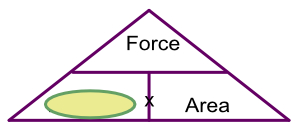Pressure

## Pressure

Pressure is a force pressing on a substance: this may be due to a weight on a floor, a tyre on the road or a mould pressing a shape.

Pressure is measured as a force (newtons) on an area (square metres, or square centimetres). it is most commonly written as N/m2, or Nm-2.

Pressure can be set out in a formula:

text(Pressure) = frac(text(Force))(text(Area))

## Example 1

An air-conditioning unit, exerting a pressure of 2.3 x 103 Newtons, has a footprint (area of floor it occupies) of 15m2. What is the pressure that the air-conditioning unit applies on the floor?Cover up Pressure

From the triangle, Pressure = Force over Area

Pressure = 2300 ÷ 15

= 153.3 N/m2

## Example 2

A floor has a safe working load of 200 N/m2. A machine, which occupies a floor area 4m by 2m and weighs 2054N, is to be placed on the floor. Is this safe?

From the triangle, hide Pressure: use Force over Area

= 2054 ÷ (4 x 2)

= 2054 ÷ 8

= 256.75 N/m2

This is greater than the floor is designed for, so the machine cannot go there.

Answer: No. The machine will exert 256.75N/m2